# forceR

## Introduction

The package forceR has originally been written for insect bite force data preparation and analysis, but it can be used for any kind of time series measurements. Functions include

• correction of charge amplifier drifts
• correction of baseline drifts
• reduction of sampling frequency
• automatic extraction of single peaks
• rescaling (normalization) of curves
• reduction of curves to 100 time steps each
• finding of best polynomial fits to describe all curves

This vignette guides you through all functions of the package. A limited data set of 8 bite force measurement files is used to get the ideas across while allowing for fast calculations.

At the end of this vignette you will find a self-sufficient workflow example that runs all forceR commands after file loading and drift corrections. Instead of loading files, bite series are simulated and then analyzed.

Please cite the following publication when using the forceR package:

Rühr, PT & Blanke, A (in press): forceX and forceR: a mobile setup and R package to measure and analyze a wide range of animal closing forces. Methods in Ecology and Evolution XXX: pp.XX-XX. doi: XXX

## Installation

### Official release

You can install the official release of forceR from CRAN:

install.packages('forceR')

### Development version

The development version of forceR is available at GitHub:

require(devtools)
devtools::install_github("https://github.com/Peter-T-Ruehr/forceR")

### Load packages to work with this vignette, including forceR

library(magrittr)
library(dplyr)
library(stringr)
library(purrr)
library(ggplot2)

library(forceR)

From hereon, we will use data stored in a local folder. Please download and unzip the content of the example_data.zip file from Zenodo onto your hard drive, unzip the files and store the folder location in the variable data.folder. This folder now contains every file that is needed and has been produced during the creation of this vignette, so you can always restore the files from Zenodo in case you have accidentally overwritten anything you wanted to keep.

If you decide to use your own files, consider that forceR generally expects file names to start with leading digits specifying the measurement number (E.g. 0001_G_maculatus.csv). The number (in this case 0001) is used to keep data files, log files, and PDF files of the same measurement associated with each other. If not already the case, we strongly recommend renaming the raw data files accordingly before continuing with this forceR vignette.

First, we set the folder containing the example data as the data.folder (make sure this fits your local path, e.g. "./example_data"):

data.folder <- "./example_data"

In case you want to use your own measurements, and if they were recorded by the LJStream software (LabJack Corporation, Lakewood, Colorado, US; tested for v1.19) as suggested in the forceX instructions, you can use the convert_measurement() function of forceR to convert your files and save them in the data.folder. See ?convert_measurement() for details.

### Plot raw measurement

The measurement file should have the time steps (preferably in m.secs, since all code of this package expects this) in the first column, and the measurements in the second column. Column names do not matter, and everything but the first two columns will be ignored.

file <- file.path(data.folder, "0982.csv")
plot_measurement(file,
columns = c(1:2))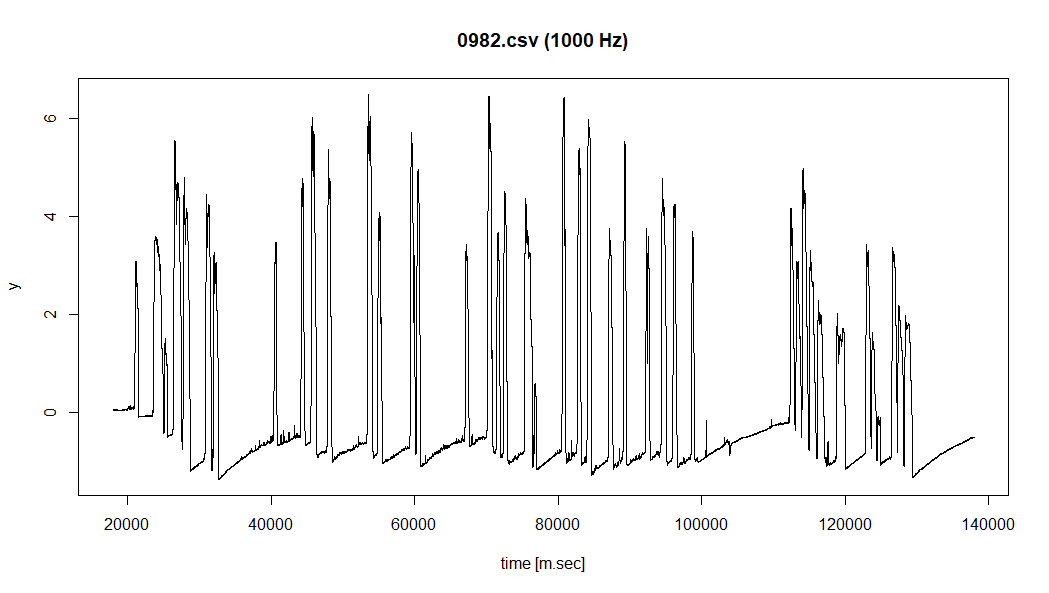### Crop raw measurement

The function crop_measurement() can be used to crop a measurement. Since we want to work with the cropped file later, we define path.data when calling the function to store the result as a new file. Before running the function, create the folder on your hard drive, e.g. at "./example_data/cropped".

cropped.folder <- "./example_data/cropped"

If a measurement contains two distinct regions of bites with a lot of unnecessary data and/or measurement artefacts in-between (such as user-made peaks), I recommend to manually copy the RAW data files, give the copy a new measurement number (as if it was actually a separate measurement), and crop the distinct parts containing actual bites separately from the two copies of the file. For more distinct regions, create more copies.

I recommend to not crop the files too much in case baseline corrections are needed later, because then the baseline_corr() function will not be able to figure out where the actual baseline might be. Leaving several seconds before and after the first and last bite of a series will prevent such problems.

file.cropped <- crop_measurement(file,
path.data = cropped.folder)

Select two points at the beginning and end of the part of the measurement we want to keep. If more than two points are selected, only the last two points will be considered. This allows for corrections of erroneous clicks. The position of the points on the y-axis is irrelevant. Make sure that the start and end points are placed on the graph between peaks, i.e. on the baseline. The y-values of the graph at these positions will be used as reference points in the following amp_drift_corr() function.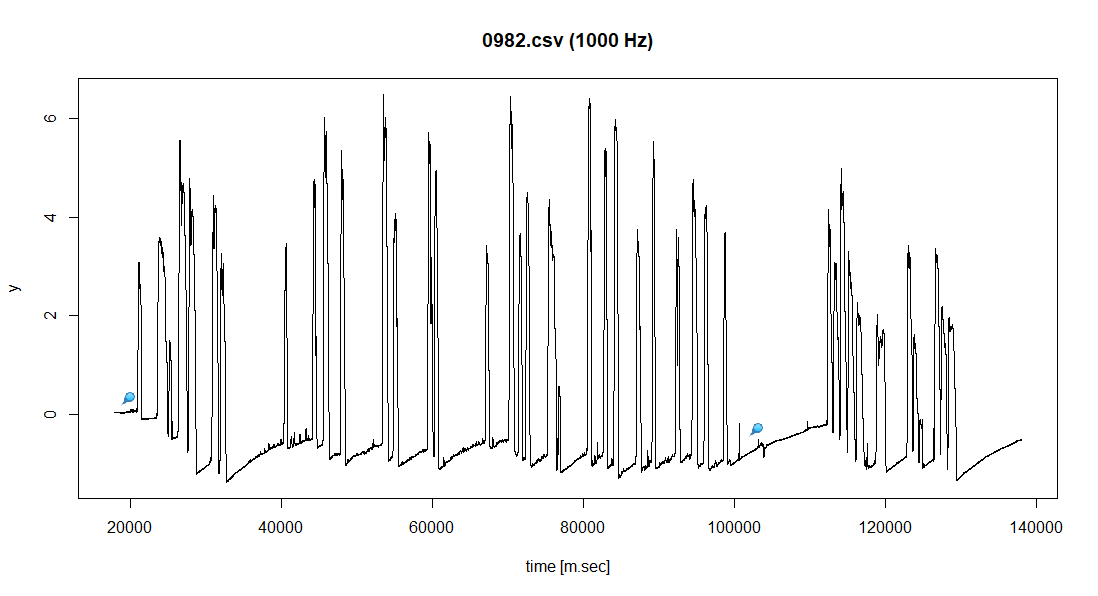The resulting file will be saved with the suffix "_cropped". We can plot it again:

cropped.folder <- "./example_data/cropped"

file <- file.path(cropped.folder, "0982_cropped.csv")
plot_measurement(file)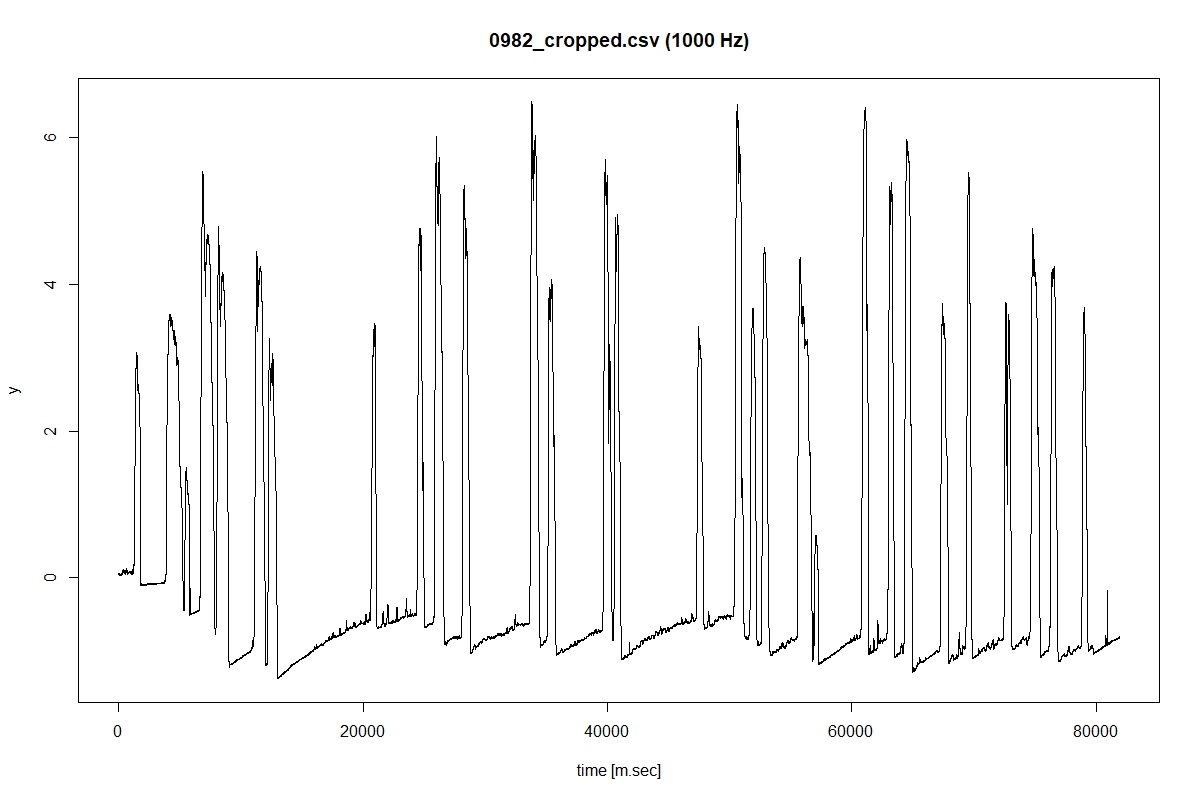### Amplifier drift correction

To remove the systemic, asymptotical drift characteristic for charge amplifiers with resistor–capacitor (RC) circuits, we can use the amp_drift_corr() function. This function requires a few parameters. ?amp_drift_corr brings up the full explanation of all values.

Basically, we only have to decide how we want to plot the data, define the correct folder with our measurement data, provide the time constant (tau, $$\tau$$) of our charge amplifier, and define a folder where we want to store the results. This function, in contrast to the previous functions, takes a folder as input because when the same charge amplifier was used for all measurements, the same settings can be used for all measurement files.

# create file list with all cropped files that need amplifier drift corrections.
#   Her we use the cropped.folder with the cropped measurements.
file.list <- list.files(cropped.folder,
pattern = "csv$", full.names = TRUE) # define folder where to save the amplifier drift corrected file. # If this folder exists, it will be created. ampdriftcorr.folder <- "./cropped/ampdriftcorr" for(filename in file.list){ print(filename) amp_drift_corr(filename = filename, tau = 9400, res.reduction = 10, plot.to.screen = FALSE, write.data = TRUE, write.PDFs = TRUE, write.logs = TRUE, output.folder = ampdriftcorr.folder, show.progress = FALSE) print("***********") } Because we set the arguments write.data, write.PDFs and write.logs to TRUE, this creates the output.folder ("./ampdriftcorr") and two new folders inside the output.folder: • "./ampdriftcorr/logs/" where log files containing info on the amp_drift_corr used are stored • "./ampdriftcorr/pdfs/" where the PDFs of the time series before and after the corrections are stored. The resulting graph of one corrected measurement may look like this: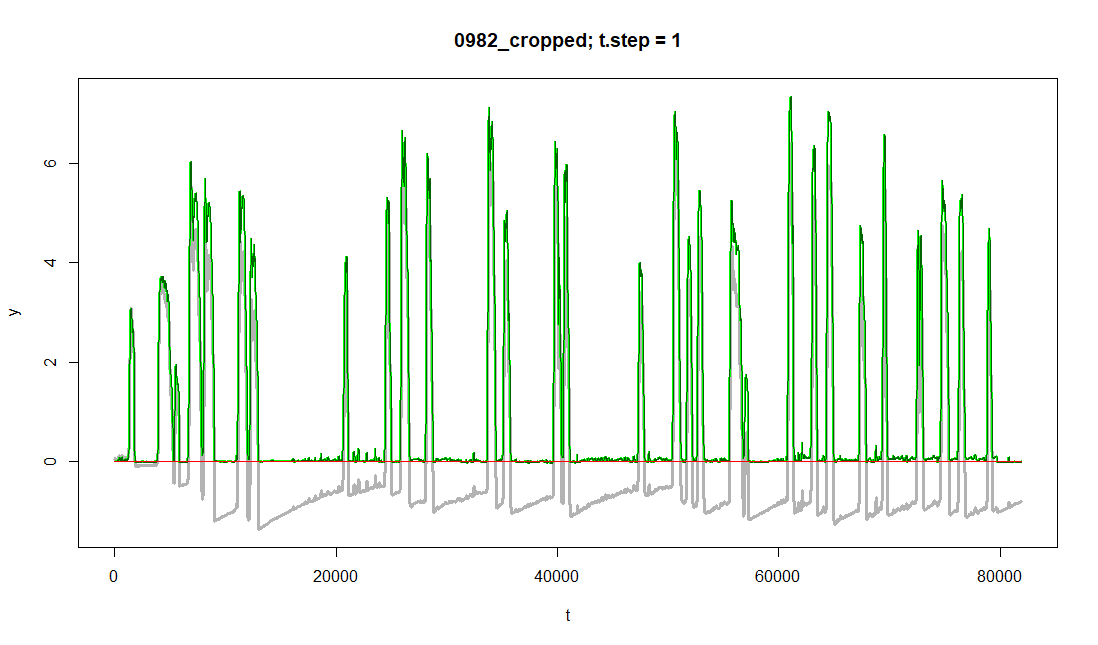In gray, we see the raw measurements, and in green the corrected data. A linear drift in the corrected data may occur in case the first value of the measurement is not exactly 0. This drift is also corrected for by the function. The final result for each file is, since write.data was set to TRUE, also saved in a new CSV file in the "./ampdriftcorr/" folder.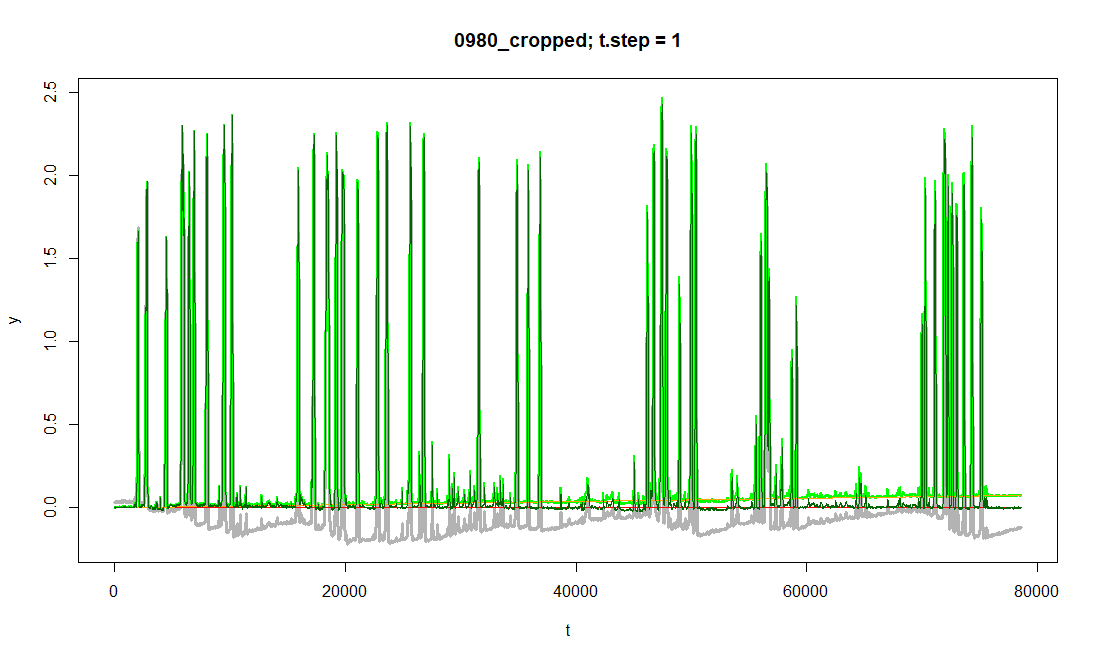### Automatic or manual baseline correction of time series If the baseline (zero-line) of a measurement is unstable (e.g. due to temperature fluctuations, wind, …), it needs to be continually adjusted throughout the measurement. Ideally, this is done automatically by the function baseline.corr(). This automatic adjustment of the baseline invokes a sliding window approach, during which the ‘minimum’ within each window is stored. A ‘minimum’ is defined by the parameter quantile.size. If quantile.size is set to 0.05, the value below which 5% of the measurement data within the current window lies, is treated as the current window’s ‘minimum’. Not taking the actual minimum within each window prevents the treatment of short undershoots (artifacts created during the measurement) as minima. In a second iteration, another sliding window calculates the average of the ‘minima’ within each window. The resulting ‘minimal’ values are subtracted from the original time series. This approach works well for time series with relatively short peaks. For longer peaks, the window.size.mins should be increased. However, the greater window.size.mins gets, the smaller becomes the baseline-correcting effect of the function. For the file "./example_data/cropped/ampdriftcorr/1068_ampdriftcorr.csv", we choose a window.size.mins of 2000:  filename = file.path(ampdriftcorr.folder, "1068_ampdriftcorr.csv") plot_measurement(filename) baselinecorr.folder <- "./cropped/ampdriftcorr/baselinecorr" file.baselinecorr <- baseline_corr(filename = filename, corr.type = "auto", window.size.mins = 2000, window.size.means = NULL, quantile.size = 0.05, y.scale = 0.5, res.reduction = 10, Hz = 100, plot.to.screen = TRUE, write.data = TRUE, write.PDFs = TRUE, write.logs = TRUE, output.folder = baselinecorr.folder, show.progress = FALSE) Again, new folders, PDFs and log files are created. The resulting plot shows the original data in gray, the ‘minima’ of the sliding windows in blue, the sliding mean of the ‘minima’ in bright green and the corrected time series in dark green: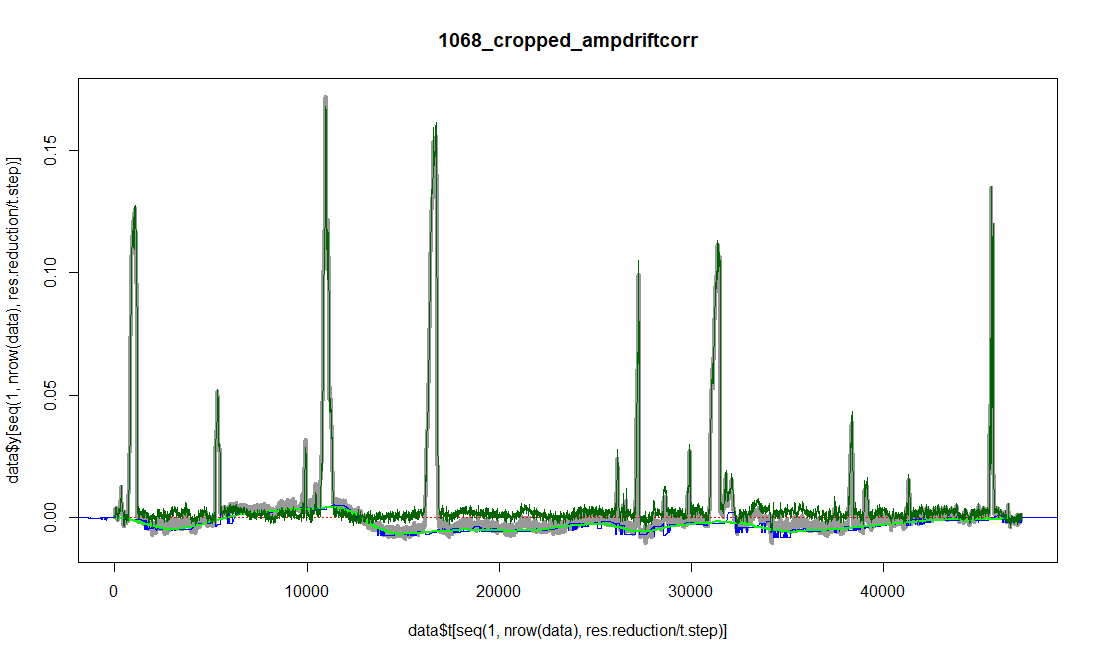If the automatic approach does not yield acceptable results, an interactive manual approach to correct the baseline can be performed instead. We do the manual correction for the file "./example_data/cropped/ampdriftcorr/1174_ampdriftcorr.csv", which contains measurements with long, plateau-like peaks where the automatic baseline correction would fail. filename = file.path(ampdriftcorr.folder, "1174_ampdriftcorr.csv") plot_measurement(file) file.baselinecorr <- baseline_corr(filename = filename, corr.type = "manual", plot.to.screen = TRUE, write.data = TRUE, write.PDFs = TRUE, write.logs = TRUE, output.folder = baselinecorr.folder, show.progress = FALSE)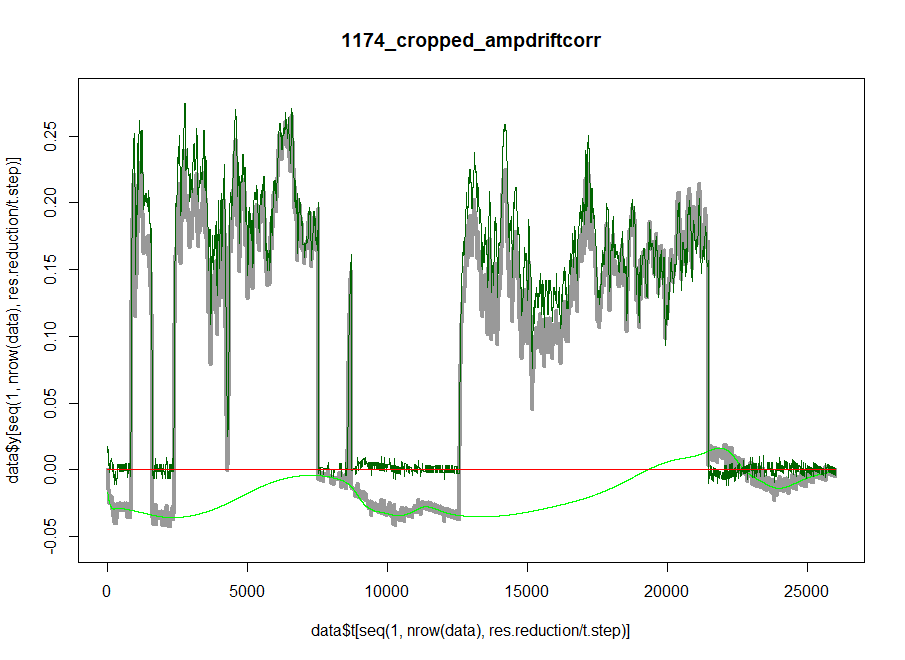The green line represents a spline along the manually defined points and is subtracted from the original data (gray). The corrected data is plotted in dark green again. We have used baseline_corr() on the files 1041_ampdriftcorr.csv, 1068_ampdriftcorr.csv, 1174_ampdriftcorr.csv, 1440_ampdriftcorr.csv. ### File sorting After all original files have been processed, we need to separate the resulting files that we want to keep from files that have been created along the way. The function sort_files() looks through a vector of folders (data.folders) to identify files with the same measurement name (i.e., the same character string in the file names before the first underscore (_) and keeps the version of the file that is found in the folder that is most closely to the end of the list of data.folders. Thus, it is important that the folders are parsed in the correct order. In our example, this order would look like this: 1. "./example_data/" 2. "./example_data/cropped/" 3. "./example_data/cropped/ampdriftcorr/" 4. "./example_data/cropped/ampdriftcorr/baselinecorr" If a file is present in "./example_data/cropped/ampdriftcorr/baselinecorr", this will be copied (move = FALSE) or moved (move = TRUE) to a folder specified in path.corrected, e.g., "./example_data/corrected/". Versions of the same measurement in the other folders will be ingored. This is iteratively repeated for the rest of the folders in reverse order of the data.folders vector. data.folders <- c(data.folder, file.path(data.folder, "/cropped"), file.path(data.folder, "/cropped/ampdriftcorr"), file.path(data.folder, "/cropped/ampdriftcorr/baselinecorr")) results.folder <- file.path(data.folder, "/corrected/") sort_files(data.folders = data.folders, results.folder = results.folder, move = FALSE) ### File loading Now we will load the first file in the results folder using load_single(). The function removes all columns except the one defined in columns = c(1:2) and renames them to t and y. Then, it adds a filename column based on the file name of the data. file.list <- list.files(results.folder, pattern = "csv", full.names = TRUE) df.1 <- load_single(file = file.list, columns = c(1:2)) df.1 #> # A tibble: 129,001 x 3 #> t y filename #> <dbl> <dbl> <chr> #> 1 0 0.00447 0979_ampdriftcorr #> 2 1 0.00954 0979_ampdriftcorr #> 3 2 0.0146 0979_ampdriftcorr #> 4 3 0.0146 0979_ampdriftcorr #> 5 4 0.00951 0979_ampdriftcorr #> 6 5 0.00951 0979_ampdriftcorr #> 7 6 0.00950 0979_ampdriftcorr #> 8 7 0.00949 0979_ampdriftcorr #> 9 8 0.00948 0979_ampdriftcorr #> 10 9 0.00948 0979_ampdriftcorr #> # ... with 128,991 more rows unique(df.1$filename)
#>  "0979_ampdriftcorr"

Alternatively, We can rapidly load all files in the results folder by using load_mult():

df.all <- load_mult(folder = results.folder,
columns = c(1:2),
show.progress = TRUE)
df.all
#> # A tibble: 615,724 x 3
#>        t       y filename
#>    <dbl>   <dbl> <chr>
#>  1     0 0.00447 0979_ampdriftcorr
#>  2     1 0.00954 0979_ampdriftcorr
#>  3     2 0.0146  0979_ampdriftcorr
#>  4     3 0.0146  0979_ampdriftcorr
#>  5     4 0.00951 0979_ampdriftcorr
#>  6     5 0.00951 0979_ampdriftcorr
#>  7     6 0.00950 0979_ampdriftcorr
#>  8     7 0.00949 0979_ampdriftcorr
#>  9     8 0.00948 0979_ampdriftcorr
#> 10     9 0.00948 0979_ampdriftcorr
#> # ... with 615,714 more rows

unique(df.all$filename) #>  "0979_ampdriftcorr" #>  "0980_ampdriftcorr" #>  "0981_ampdriftcorr" #>  "0982_ampdriftcorr" #>  "1041_baselinecorr" #>  "1068_baselinecorr" #>  "1174_baselinecorr" #>  "1440_baselinecorr"  The new tibble contains the measurement of all files in the selected folder. ## Detailed example workflow: Data analysis To allow users to test the package and follow the analysis steps of this vignette without having to load actual files, we have simulated data using the forceR function simulate_bites() and stored them within forceR. We will replace our previous df.all tibble with the internal forceR tibble. You may, however, continue with your just created version of df.all. # create/replace df.all df.all <- forceR::df.all head(df.all) #> # A tibble: 6 x 3 #> t y measurement #> <int> <dbl> <chr> #> 1 1 0 m_01 #> 2 2 0 m_01 #> 3 3 0 m_01 #> 4 4 0 m_01 #> 5 5 0 m_01 #> 6 6 0 m_01 Now let us plot the simulated measurements ot get a feeling for them: # plot simulated measurements ggplot(df.all, aes(x = t , y = y, colour=measurement)) + geom_line()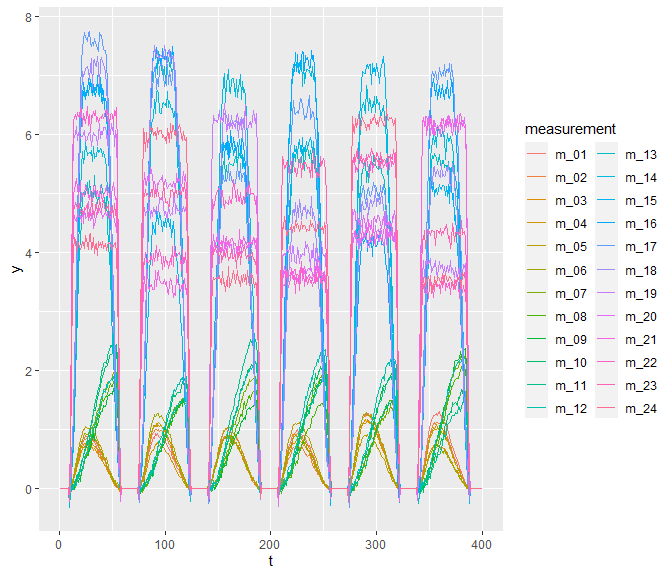We can see that some bites have more (reddish colors) or less (blueish colors) plateau-like peaks, whereas others have more sinusoidal peaks with early (orange) or late peaks (green). ### Sampling Frequency Reduction df.all has a sampling frequency of 1000 Hz. This is more than is often needed to analyse a time series. To reduce the sampling frequency of all measurements that are above a desired frequency of e.g. 200 Hz, we can use the function reduce.frq(). However, please not that the simulated bites are only 50 ms long, which is much shorter than, e.g., actual insect bites. This will keep all calculations quick, but will result in measurement series that are more downsampled than we would recommend. For real insect bites, though, a sampling frequency of 200 Hz is sufficient, so we will proceed as if we were working with such real bites: # reduce frequency to 200 Hz df.all.200 <- reduce_frq(df = df.all, Hz = 200, measurement.col = "measurement") head(df.all.200) #> # A tibble: 6 x 3 #> # Groups: t  #> t y measurement #> <dbl> <dbl> <chr> #> 1 0 0 m_01 #> 2 5 0 m_01 #> 3 10 0.0439 m_01 #> 4 15 0.299 m_01 #> 5 20 0.644 m_01 #> 6 25 0.821 m_01 If measurement.col is not defined, the whole input data frame will be treated as if it was just one single time series. This is okay for data frames like df.1 that only contain one measurement, but for data frames with multiple time series measurements (like df.all), we need to define a grouping column - in our case the column measurement. ### Creating a classifier with dataset info For further analyses, we need a classifier in which data on the species, specimens and measurements of our dataset are stored: # create a classifier number_of_species <- 4 number_of_specimens_per_species <- 3 number_of_measurements_per_specimen <- 2 number_of_rows <- number_of_species * number_of_specimens_per_species * number_of_measurements_per_specimen species <- sort(rep(paste0("species_", LETTERS[1:number_of_species]), length=number_of_rows)) specimens <- sort(rep(paste0("speciemen_", letters[1:(number_of_species*number_of_specimens_per_species)]), length=number_of_rows)) classifier <- tibble(species = species, specimen = specimens, measurement = paste0("m_", str_pad(string= 1:number_of_rows, width = 2, pad = "0")), amp = c(rep(0.5, number_of_rows/2), rep(2, number_of_rows/2)), lever.ratio = rep(0.5, number_of_rows)) head(classifier) #> # A tibble: 6 x 5 #> species specimen measurement amp lever.ratio #> <chr> <chr> <chr> <dbl> <dbl> #> 1 species_A speciemen_a m_01 0.5 0.5 #> 2 species_A speciemen_a m_02 0.5 0.5 #> 3 species_A speciemen_b m_03 0.5 0.5 #> 4 species_A speciemen_b m_04 0.5 0.5 #> 5 species_A speciemen_c m_05 0.5 0.5 #> 6 species_A speciemen_c m_06 0.5 0.5 ### Convert measurement to force To convert a voltage measurement to a force measurement [Newton], we need an amplification value and, depending on the measurement setup, the lever ratio of the lever forwarding the force from the force plate to the sensor. Now we can convert the y-values of df.all.200 to force data and add the specimen and species info from the classifier using the y_to_force() function: df.all.200.tax <- y_to_force(df = df.all.200, classifier = classifier, measurement.col = "measurement") head(df.all.200.tax) #> # A tibble: 6 x 4 #> # Groups: t  #> measurement specimen t force #> <chr> <chr> <dbl> <dbl> #> 1 m_01 speciemen_a 0 0 #> 2 m_01 speciemen_a 5 0 #> 3 m_01 speciemen_a 10 0.0439 #> 4 m_01 speciemen_a 15 0.299 #> 5 m_01 speciemen_a 20 0.644 #> 6 m_01 speciemen_a 25 0.821 ### Minimum, maximum and standard deviation of force data per measurement and species #### Initial reduction to one row per specimen Finally, we can start with the actual analysis of the data. First, we want to calculate the minimum, maximum and standard deviation of force for each measurement and, in case there were several measurements per specimen, also for each specimen. From the previous steps, df.all.200.tax already contains the columns measurement, specimen and species. Now, we can use the function summarize_measurements() to create a summary tibble by adding the column names for which we want a summary in var1 and var2. var1 must name the column that stores the unique measurement IDs, e.g. measurement number, to calculate minimal and maximal force values per measurement. As var2 we will use ‘specimen’ to calculate the summary data per specimen from the min. and max. values of the measurements of that specimen. Thus, the resulting tibble will contain summaries of all measurements that were performed with the same specimen. # add species to df.all.200.tax df.all.200.tax <- df.all.200.tax %>% left_join(classifier %>% select(species, measurement)) var1 = "measurement" var2 = "specimen" df.summary.specimen <- summarize_measurements(df.all.200.tax, var1, var2) head(df.summary.specimen) #> # A tibble: 6 x 8 #> measurement specimen species max.F.measureme~ mean.F.specimen max.F.specimen #> <chr> <chr> <chr> <dbl> <dbl> <dbl> #> 1 m_01 specieme~ species~ 1.24 1.24 1.24 #> 2 m_02 specieme~ species~ 1.24 1.24 1.24 #> 3 m_03 specieme~ species~ 1.13 1.13 1.13 #> 4 m_04 specieme~ species~ 1.12 1.13 1.13 #> 5 m_05 specieme~ species~ 1.09 1.18 1.26 #> 6 m_06 specieme~ species~ 1.26 1.18 1.26 #> # ... with 2 more variables: sdv.max.F.specimen <dbl>, #> # n.measurements.in.specimen <int> # boxplot of maximum force in specimens ggplot(data = df.summary.specimen, mapping = aes(x=specimen,y=max.F.measurement)) + geom_jitter(aes(color='blue'),alpha=0.7, width = 0.2, height = 0.0) + geom_boxplot(fill="bisque",color="black",alpha=0.3) + # scale_y_log10() + labs(y="max(F)/specimen") + guides(color="none") + theme_minimal() + theme(axis.text.x = element_text(angle = 45, hjust=1))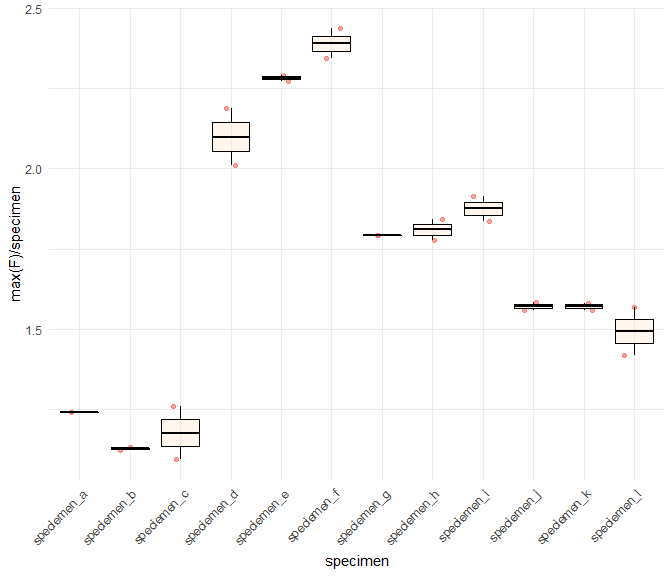#### Further summary calculations for species-wise info To get a summary on species-wise info, we have to calculate with the summarized specimen info. We are not using the summarize_measurements() functions because this would ignore the fact the some measurements of one species may come from the same specimen, but we only want to consider one maximum force value per specimen and not one per measurement. df.summary.species <- df.summary.specimen %>% # find max Fs of species group_by(species) %>% # calculate force values for each species mutate(max.F.species = max(max.F.specimen), mean.F.species = round(mean(max.F.specimen),6), sdv.max.F.species = sd(max.F.specimen)) %>% ungroup() %>% # count specimens / species group_by(species) %>% mutate(n.specimens.in.species = length(unique(specimen))) %>% ungroup() df.summary.species #> # A tibble: 24 x 12 #> measurement specimen species max.F.measureme~ mean.F.specimen max.F.specimen #> <chr> <chr> <chr> <dbl> <dbl> <dbl> #> 1 m_01 specieme~ specie~ 1.24 1.24 1.24 #> 2 m_02 specieme~ specie~ 1.24 1.24 1.24 #> 3 m_03 specieme~ specie~ 1.13 1.13 1.13 #> 4 m_04 specieme~ specie~ 1.12 1.13 1.13 #> 5 m_05 specieme~ specie~ 1.09 1.18 1.26 #> 6 m_06 specieme~ specie~ 1.26 1.18 1.26 #> 7 m_07 specieme~ specie~ 2.19 2.10 2.19 #> 8 m_08 specieme~ specie~ 2.01 2.10 2.19 #> 9 m_09 specieme~ specie~ 2.27 2.28 2.29 #> 10 m_10 specieme~ specie~ 2.29 2.28 2.29 #> # ... with 14 more rows, and 6 more variables: sdv.max.F.specimen <dbl>, #> # n.measurements.in.specimen <int>, max.F.species <dbl>, #> # mean.F.species <dbl>, sdv.max.F.species <dbl>, n.specimens.in.species <int> # boxplot of maximum force in species ggplot(data = df.summary.species, mapping = aes(x=species,y=max.F.specimen)) + geom_jitter(aes(color='blue'),alpha=0.7, width = 0.2, height = 0.0) + geom_boxplot(fill="bisque",color="black",alpha=0.3) + # scale_y_log10() + labs(x='species', y="max(F)/specimen") + guides(color="none") + theme_minimal()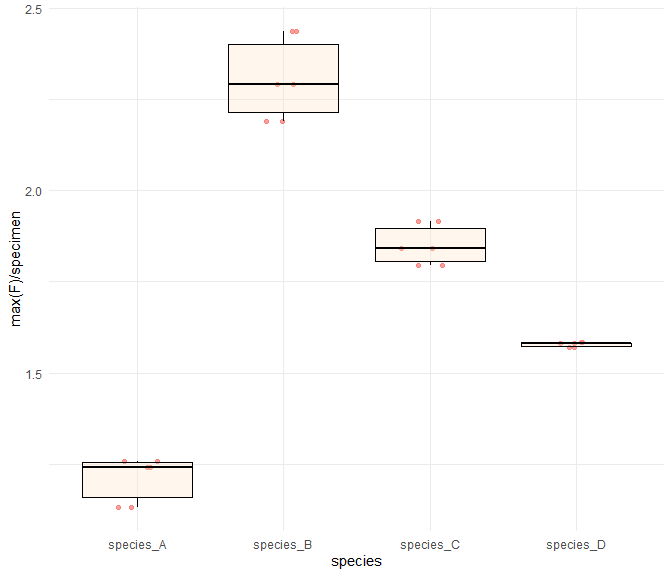### Identify bites Now we come to one of the core functions of forceR: find_strongest_peaks(). This function identifies the strongest peaks of each measurement. But first, we need to create some folders to store the data and plots that are produced in all subsequent analyses of this example: # create folders to save df and results path.plots <- paste0(data.folder, "/plots/") ifelse(!dir.exists(path.plots), dir.create(path.plots), "./plots already exists") path.plots.initial_peak_finding <- paste0(data.folder, "/plots/initial_peak_finding/") ifelse(!dir.exists(path.plots.initial_peak_finding), dir.create(path.plots), "./plots/initial_peak_finding already exists") path.data <- paste0(data.folder, "/data/") ifelse(!dir.exists(path.data), dir.create(path.data), "./data already exists") Now we let find_strongest_peaks() identify all actual peak curves in all measurements: peaks.df <- find_strongest_peaks(df = df.all.200.tax, no.of.peaks = 5, path.data = path.data, path.plots = path.plots, show.progress = TRUE) head(peaks.df) #> # A tibble: 4 x 4 #> species measurements starts ends #> <chr> <chr> <chr> <chr> #> 1 species_A m_06; m_01; m_02; m_01; m_06 265; 265; 265; 335; 70 325; 325; 325; ~ #> 2 species_B m_11; m_11; m_12; m_10; m_09 140; 5; 205; 5; 340 200; 65; 265; 6~ #> 3 species_C m_17; m_16; m_18; m_16; m_18 5; 70; 70; 200; 5 65; 130; 130; 2~ #> 4 species_D m_19; m_22; m_24; m_21; m_20 135; 0; 265; 330; 330 200; 65; 330; 3~ The initial peak finding in the first iteration of the function find_strongest_peaks() identifies peaks via a the initial.threshold which is by default set to 0.05 (= 5%) of the maximum force of the respective measurement. The threshold is indicated as a green line in the plots. Bite starts are indicated as blue points, peak ends as orange points: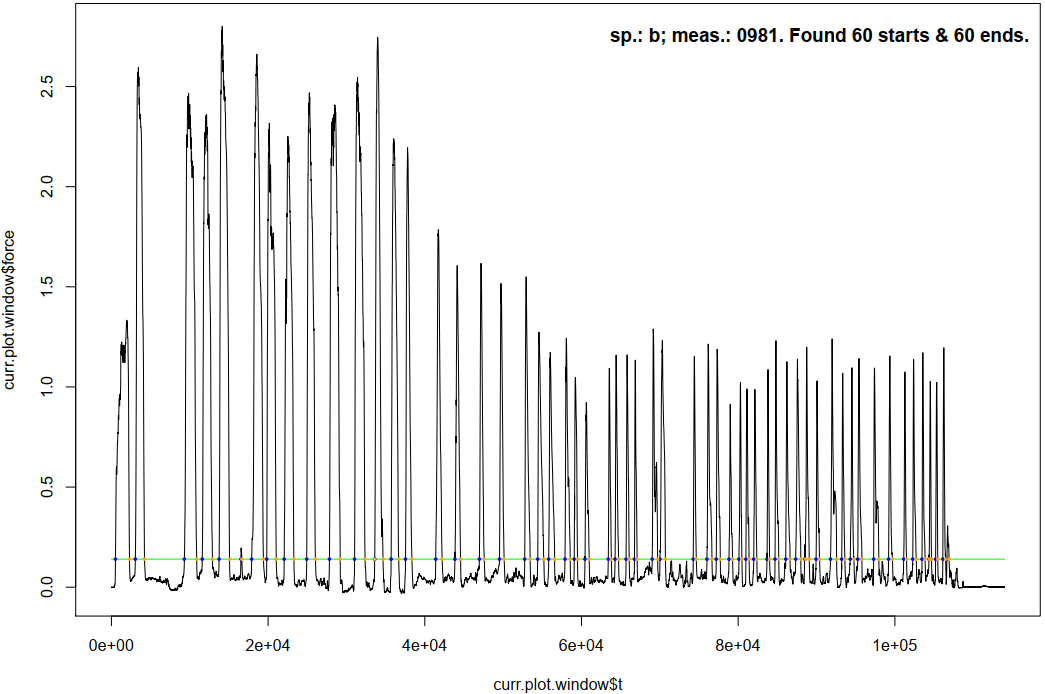In a second iteration, the function find_strongest_peaks() optimizes the peak starts and ends found in the first iteration. Starting from each start, a sliding window of size slope.length.start (in time steps) moves backwards in time and calculates the slope within the current window. The parameter slope.thresh.start defines below which slope the process is stopped. The time point where the threshold is reached is treated as the actual start of that peak. The same is done in forward direction with the peak ends. Here, slope.length.end (in time steps) defines the sliding window size, and slope.thresh.end defines the slope threshold. All these settings have default values which proved as good choices for insect bites. The peak optimization will not be done for all peaks, but only for the strongest peaks per species (not per measurement!), specified by no.of.peaks. If fewer than no.of.peaks peaks are found for a species, the results table will contain fewer peaks for this species. No warning is issued. The results are not automatically plotted. This can be done with the function plot_peaks(): plot_peaks(df.peaks = peaks.df, df.data = df.all.200.tax, additional.msecs = 2000, path.plots = path.plots) The first page of the resulting PDF looks like this, showing the five strongest peaks of species_A, which was measured in measurements m_01 - m_06, and the strongest peak of species_A which occured in measurement m_11. Plots of further peaks of species_A and the peaks of the other species follow on the next pages.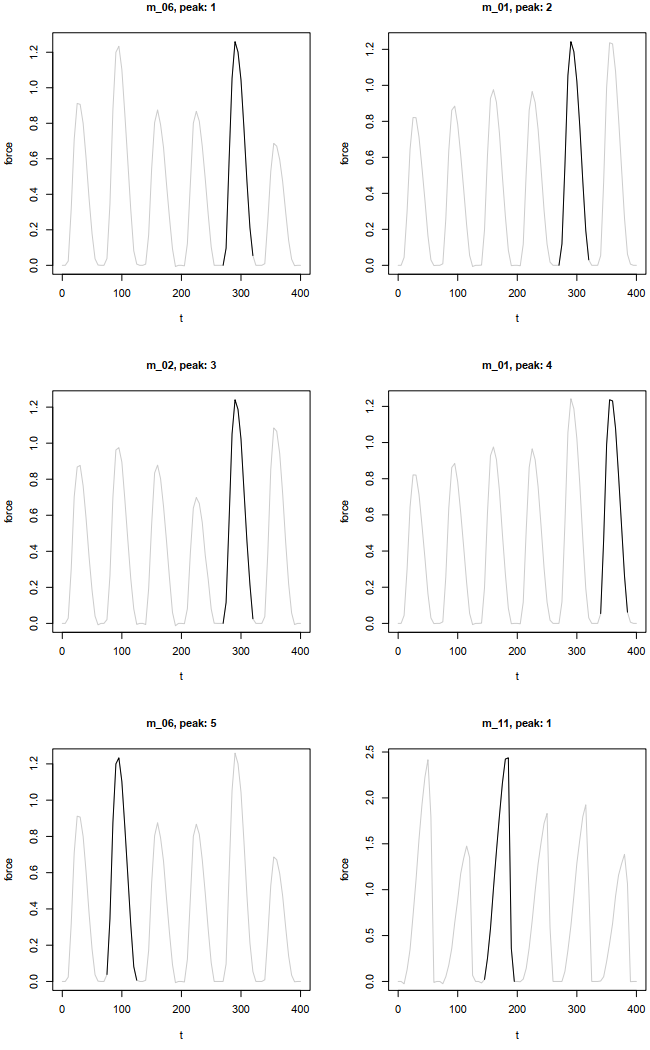We strongly recommend saving df.peaks as a csv file on your computer and keep a copy of it save. The following procedures overwrite values in this table, so the peak-finding step needs to be repeated to restore original values. ### Manual peak corrections For some measurements, the automatic finding identification of peak starts and ends may fail. Changing the parameters of find_strongest_peaks() might help, but in some cases, the starts and ends have to be manually defined. For these cases, the function correct_peak() has been written. Set path.data to the folder where you stored peaks.df before. This will overwrite the info of the bite you want to correct (here: bite 1 of measurement m_01! peaks.df <- correct_peak(df.peaks = peaks.df, df.data = df.all.200.tax, measurement = "m_01", peak = 1, additional.msecs = 100, path.data = path.data)  "Select new peak start and end. If more than two points are selected, the operation is automatically terminated." This functions prompts the user to define a new start and end of the peak. Define the measurement ID (as a character string) in which the peak to correct is located, and the peak number (as a numerical value). Both can be found in the respective title of the peak curve plot created by plot_peaks(). If the desired start and/or end lies outside of the plot window, increase additional.msecs.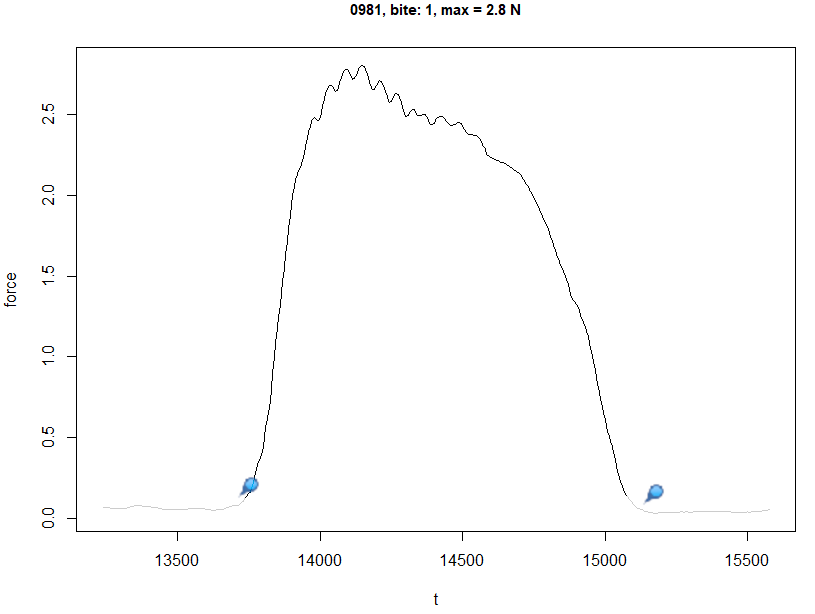The function overwrites the start and end values of the selected peak in the selected measurement and plots the corrected peak curve. Again, note that this example is taken from real measurements and not the simulated data we use in the other analysis steps.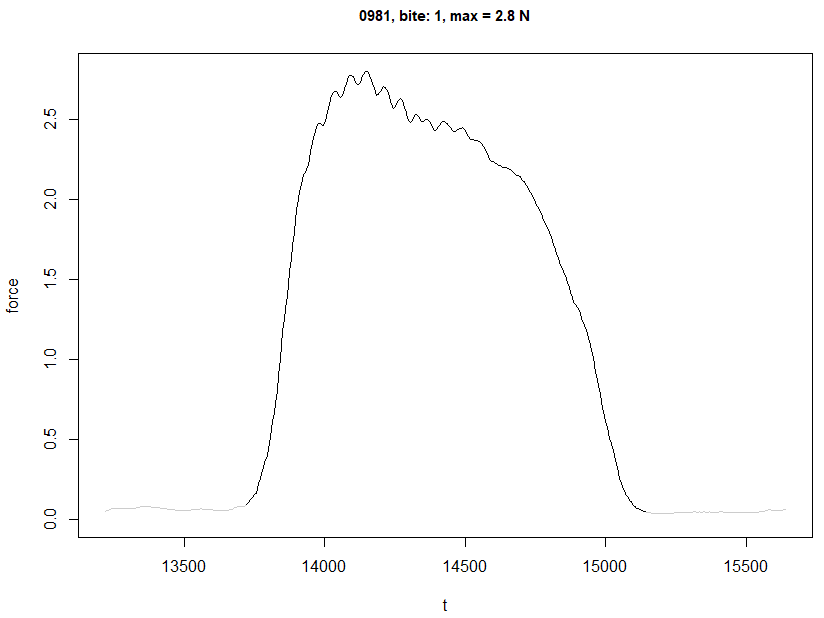Note that the peak in the figures above looks differently from the one in your plots, but the principles are the same. ### Normalize (rescale) peaks In the next step, we will use rescale_peaks() to rescale the time and force data so that they both range from 0 to 1. This function takes the data frame that contains the individual peak starts and ends, and the measurement data. Both data frames are linked by the ‘measurement’ column, so this column needs to be present in both data frames. peaks.df.norm <- rescale_peaks(df.peaks = peaks.df, df.data = df.all.200.tax, plot.to.screen = TRUE, path.data = path.data, show.progress = TRUE) head(peaks.df.norm) #> # A tibble: 6 x 6 #> measurement peak t.norm force.norm specimen species #> <chr> <int> <dbl> <dbl> <chr> <chr> #> 1 m_06 1 0 0 speciemen_c species_A #> 2 m_06 1 0.0833 0 speciemen_c species_A #> 3 m_06 1 0.167 0.0764 speciemen_c species_A #> 4 m_06 1 0.25 0.467 speciemen_c species_A #> 5 m_06 1 0.333 0.836 speciemen_c species_A #> 6 m_06 1 0.417 1 speciemen_c species_A Now we will plot the resulting data. You will note that the peaks are not very smooth. This is because the simulated peaks of df.all where so short that the reduction to 200 Hz in one of the previous steps resulted in very few time steps per bite. It is, thus, important to choose the sampling frequency wisely. For our example, however, these few time steps are sufficient. # plot all normalized peaks ggplot(peaks.df.norm %>% mutate(color.column = paste0(measurement, "__bite_", peak)), aes(x = t.norm , y = force.norm, colour=color.column)) + geom_line()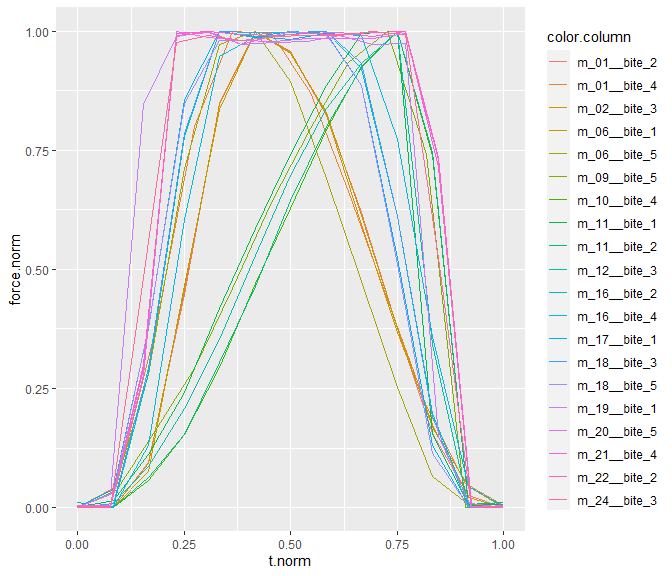### Reduce to 100 time steps per peak peaks.df.norm now contains the same data as df.all.200.tax, only with the additional columns of t.norm and force.norm which contain the rescaled time and force data, both ranging from 0 to 1. To reduce the data to 100 observations (time steps), we will use the function red_peaks_100(). Note that, because the peaks of the example dataset are so short, we now actually increase the number of time steps. In real data, however, red_peaks_100() usually boils down the number of observations. peaks.df.norm.100 <- red_peaks_100(df = peaks.df.norm, plot.to.screen = TRUE, path.data = path.data, path.plots = path.plots, show.progress = TRUE) head(peaks.df.norm.100) head(peaks.df.norm.100) #> # A tibble: 6 x 6 #> species measurement specimen peak index force.norm.100 #> <chr> <chr> <chr> <int> <dbl> <dbl> #> 1 species_A m_06 speciemen_c 1 1 0 #> 2 species_A m_06 speciemen_c 1 2 0.00568 #> 3 species_A m_06 speciemen_c 1 3 0.00891 #> 4 species_A m_06 speciemen_c 1 4 0.0101 #> 5 species_A m_06 speciemen_c 1 5 0.00972 #> 6 species_A m_06 speciemen_c 1 6 0.00814 Again, we will plot the result: head(peaks.df.norm.100) #> # A tibble: 6 x 6 #> species measurement specimen peak index force.norm.100 #> <chr> <chr> <chr> <int> <dbl> <dbl> #> 1 species_A m_06 speciemen_c 1 1 0 #> 2 species_A m_06 speciemen_c 1 2 0.00568 #> 3 species_A m_06 speciemen_c 1 3 0.00891 #> 4 species_A m_06 speciemen_c 1 4 0.0101 #> 5 species_A m_06 speciemen_c 1 5 0.00972 #> 6 species_A m_06 speciemen_c 1 6 0.00814 # plot normalized peaks: 5 bites per measurement ggplot(peaks.df.norm.100 %>% mutate(color.column = paste0(measurement, "-bite", peak)), aes(x = index , y = force.norm.100, colour=color.column)) + geom_line()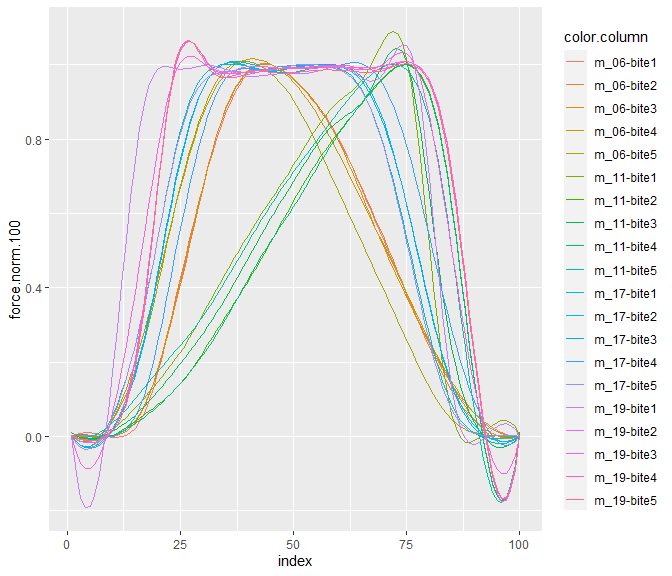The resulting tibble consists of 100 rows per peak, and within each peak, the index column runs from 1 to 100, and the force.norm.100 column consists of the rescaled peak curves, each of whose values range from 0 to 1. Since we included 4 species in our dataset, the resulting tibble is 2,000 rows long (species * peaks/species * time steps per peak = 4 * 5 * 100 = 2,000). ### Average peak curve per species Next, we will calculate the average peak curve per species and normalize the curves again: peaks.df.100.avg <- avg_peaks(df = peaks.df.norm.100, path.data = path.data) head(peaks.df.100.avg) #> # A tibble: 6 x 3 #> # Groups: species  #> species index force.norm.100.avg #> <chr> <dbl> <dbl> #> 1 species_A 1 0.00531 #> 2 species_A 2 0.00267 #> 3 species_A 3 0.000855 #> 4 species_A 4 0 #> 5 species_A 5 0.000247 #> 6 species_A 6 0.00173 # plot averaged normalized curves per species ggplot(peaks.df.100.avg, aes(x = index , y = force.norm.100.avg, colour=species)) + geom_line()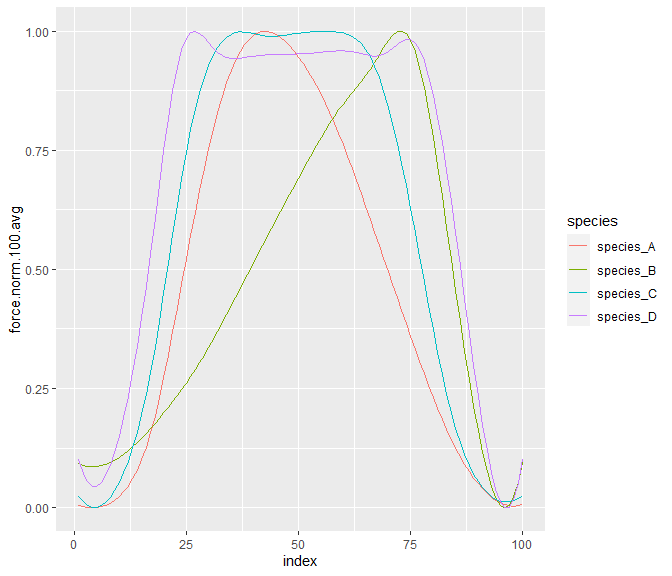### Fit polynomial functions to the force curves To find out the minimum number of coefficients that well describe all curves, we use the function find_best_fits(). It fits polynomial functions with 1 to 20 coefficients and uses the Akaike Information Criterion (AIC) to evaluate the goodness of the fits. A model is considered a good fit when the percentage of change from one model to the next (e.g. a model with 6 coefficients to a model with 7 coefficients) is <= 5%. The first four models meeting this criterion are plotted as colored graphs and the AICs of these models are visualized in a second plot for each curve. All first four coefficients per curve that fulfill the criterion are stored and in the end, a histogram of how often which coefficients were good fits is plotted as well. The function returns the numerical value of the coefficient that fulfilled the criterion of a good fit in most curves. If write.PDFs == TRUE, then the plots are saved as PDFs in path.plots. Resulting data is saved in path.data. best.fit.poly <- find_best_fits(df = peaks.df.100.avg, plot.to.screen = TRUE, path.data = path.data, path.plots = path.plots) best.fit.poly #>  10 Both 4 and 8 coefficients most often were under the first 4 models that were followed by an AIC-change below 5%: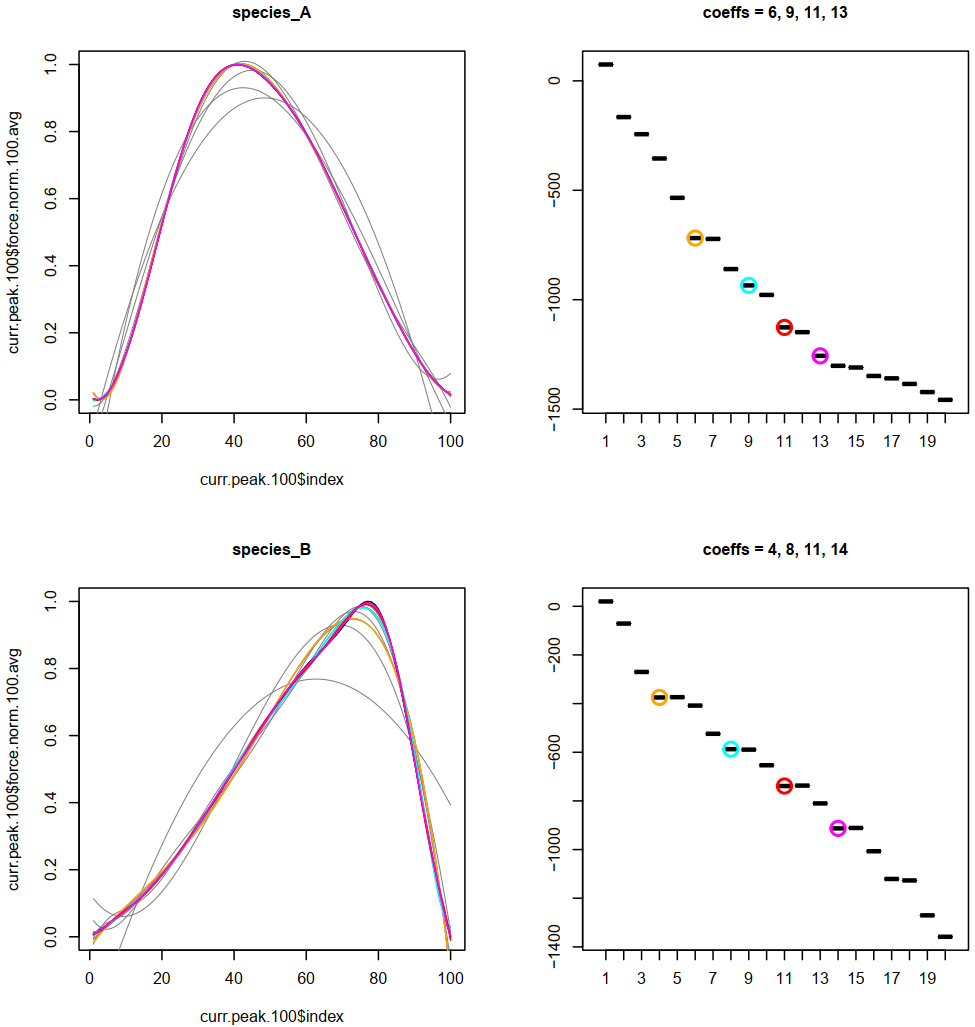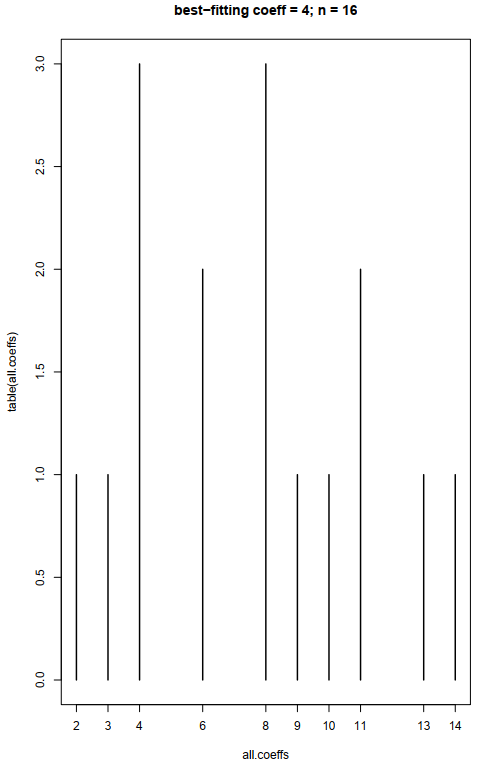The first image shows the polynomial fits to the average force curves of species_A and species_B. The second image shows a frequency distribution of how often a polynomial function with n coefficients was followed by a function with n+1 coefficients that only brought an AIC-change below 5%. Given the fact that we only analyzed four species in this example, the histogram looks a bit scarce (n = 4 species * 4 coefficients = 16). If more species are analyzed, this may rather look like this: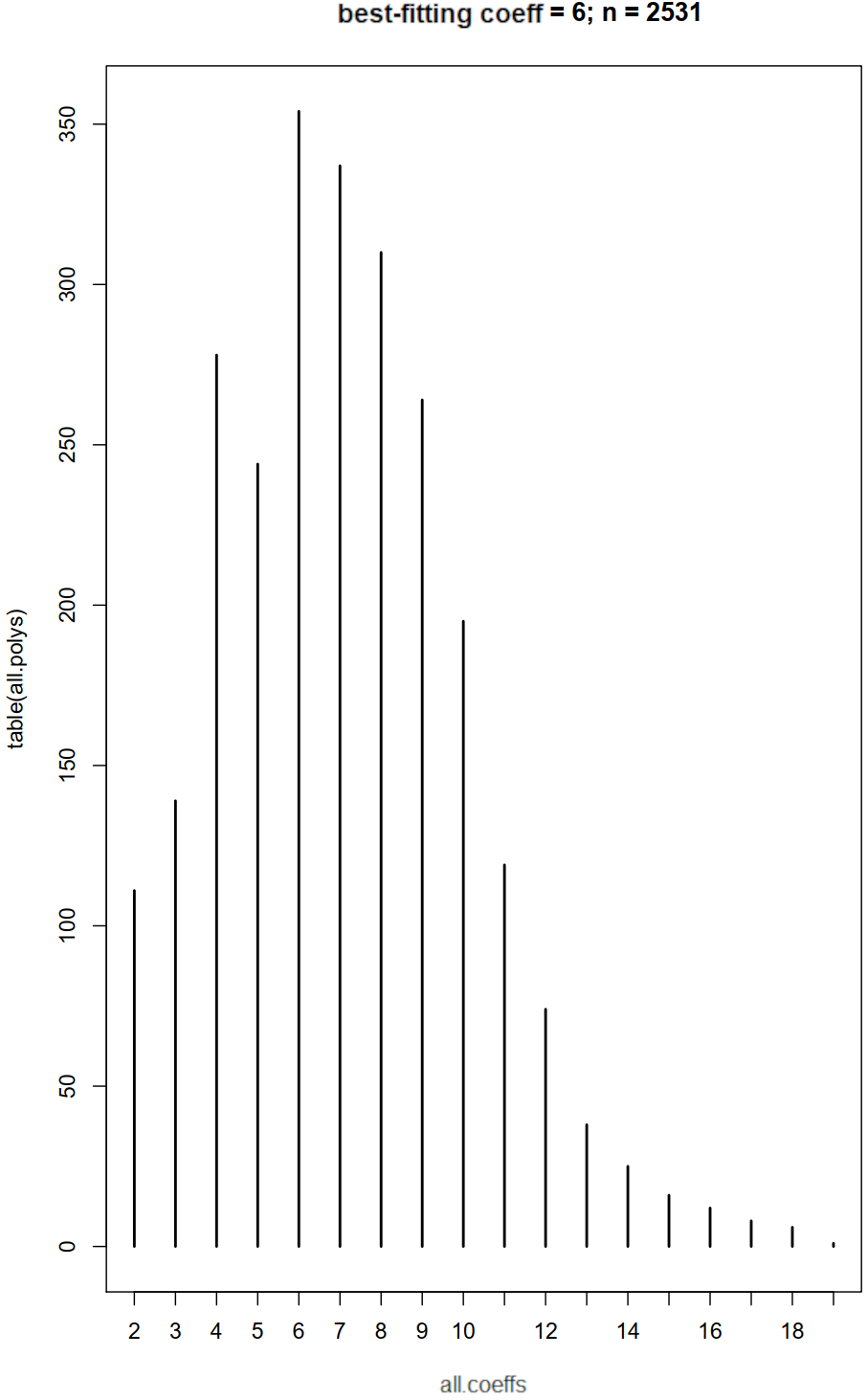#### Convert curves to polynomial models In the last step of this vignette, we convert the average peak curve shape into polynomial models in which all have the same amount of coefficients (defined by the previously identified best.fit.poly): models <- peak_to_poly(df = peaks.df.100.avg, coeff = best.fit.poly, path.data = path.data, show.progress = TRUE) # create tibble with model data models.df <- NULL for(i in 1:length(models)){ model.df <- tibble(species = rep(names(models)[i], 100), index = 1:100, y = predict(models[[i]])) models.df <- rbind(models.df, model.df) } # plot all polynomial models ggplot(models.df, aes(x = index , y = y, colour=species)) + geom_line()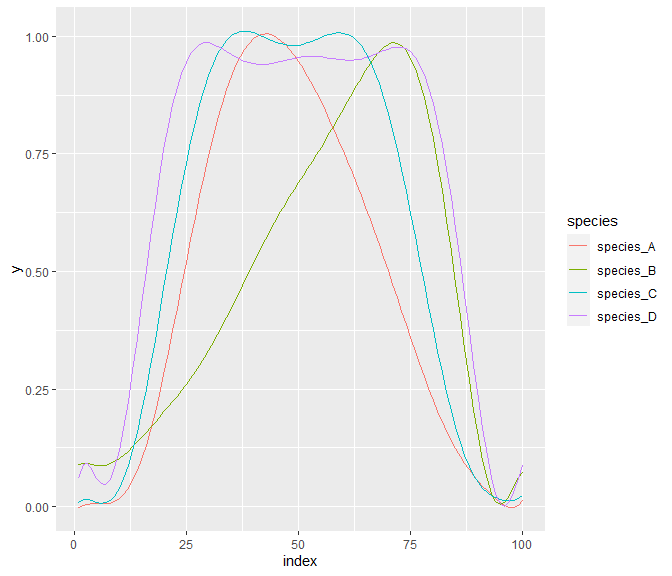## Well done! Congratulations - you have made it to the end of this vignette. We hope this package helps you with your data. In case of any remarks or questions, please feel free to contact Peter T. Rühr at peter.ruehr at gmail.com. ## forceR workflow example Below we have compiled a self-sufficient R script to run all functions of forceR after file loading and drift corrections. Instead of loading data from in vivo measurements, we simulate bite series with the function simulate_bites() and store it in df.all. The variable names used are the same as used in the rest of this vignette. They are also stored in the forceR package internally and can be restored by calling, e.g. df.all <- forceR::df.all. ## --------------------------- ## ## PURPOSE OF SCRIPT: ## Simulate data and test various functions of the forceR package. ## ## ## AUTHOR: ## Peter T. RÃ¼hr ## ## DATE CREATED: ## 2022-04-07 ## ## Copyright (c) Peter T. RÃ¼hr, 2022 ## Email: peter.ruehr@gmail.com ## ## --------------------------- ## ## NOTES: ## ## ## ## ------------------------------------------------------------------------- ## DEPENDENCIES # to use viewport() require(grid) # forceR install.packages("C:/Users/pruehr.EVOLUTION/Documents/forceR", repos = NULL, type = "source") library(forceR) # various plotting functions require(ggplot2) # load tidyverse for its various conveniences require(tidyverse) ## ------------------------------------------------------------------------- ## FUNCTIONS # get data as string for saving files today <- function(){ date.string <- gsub("-", "_", substring(as.character(as.POSIXct(Sys.time())), 1, 10)) return(date.string) } # plot linear regression and return relevant values plot.linear.regression <- function(x, y, logarithmic = F, x.axis.label = "x", title = NULL, x.lim = NULL, y.lim = NULL){ if(logarithmic == "10"){x <- log10(x); y <- log10(y)} if(logarithmic == "e"){x <- log(x); y <- log(y)} lin.mod <- lm(y ~ x) lin.mod.sum <- summary(lin.mod) lin.mod.r2 <- lin.mod.sum$adj.r.squared
lin.mod.p <- lin.mod.sum$coefficients[2,4] lin.mod.intercept <- lin.mod$coefficients
lin.mod.slope <- lin.mod$coefficients lin.mod.label.r2 <- bquote(italic(R)^2 == .(format(lin.mod.r2, digits = 3))) if(lin.mod.p > 0.05) {lin.mod.p.ast <- lin.mod.p} if(lin.mod.p <= 0.05 & lin.mod.p > 0.01) {lin.mod.p.ast <- "*"} if(lin.mod.p <= 0.01 & lin.mod.p > 0.001) {lin.mod.p.ast <- "**"} if(lin.mod.p <= 0.001) {lin.mod.p.ast <- "***"} lin.mod.label.p <- bquote(italic(p) == .(lin.mod.p.ast)) if(is.null(xlim)){ x.lim = c(min(x), max(x)) } if(is.null(ylim)){ y.lim = c(min(y), max(y)) } if(lin.mod.p >= 0.001) p.print <- paste0("p = ", round(lin.mod.p, 3)) if(lin.mod.p < 0.001) p.print <- "p < 0.001" plot(x, y, pch = 16, xlab = paste0(x.axis.label, ": ", p.print, "; R2 = ", round(lin.mod.r2,3), "; m = ", round(lin.mod.slope,3), "; y = ", round(lin.mod.intercept,3)), xlim = x.lim, ylim = y.lim) abline(lin.mod, col = "red") if(!is.null(title)){ title(main = title) } print(x.axis.label) print(paste0(p.print)) print(paste0("R2 = ", round(lin.mod.r2,3))) print(paste0("m = ", round(lin.mod.slope,3))) print(paste0("y = ", round(lin.mod.intercept,3))) res <- tibble(p = lin.mod.p, r2 = lin.mod.r2, intercept = lin.mod.intercept, slope = lin.mod.slope) return(res) } # add leading zeros to number and return as string add_eading_zeros <- function(number, length){ require(stringr) str_pad(number, length, pad = "0") } ## ------------------------------------------------------------------------- ## Simulate bite force data # set seed for randomization so results are reproducible set.seed(1) ## ------------------------------------------------------------------------- ## Create classifier to store data (1 row per measurement) classifier <- tibble(bite.type = c(rep("sinosoidal", 5), rep("plateau", 3), rep("inter", 4)), peak.position = c("early","early","center","late","late","center","center","center","center","center","early","late"), species = NA, specimen = NA, measurement = NA, body_mass = NA, force_in = NA, length.of.bite = 4000, peak.pos = c(20, 25, 50, 65, 70, rep(NA, 7)), slope.perc.starts = c(rep (NA, 5), 10,15,20,30,40,20,50), slope.perc.ends = c(rep (NA, 5), 10,15,20,30,40,50,20), type = c(rep("sin", 5), rep("plat", 7)), no.of.bites = 7, amp = 1, lever.ratio = 1, length.of.series = 35000) # amend classifier classifier <- classifier %>% mutate(no = row_number()) classifier # define maximum forces per bite simulation type and add additional rows to classifier forces <- c(1, 5, 15) for(i in 1:nrow(classifier)){ classifier$force_in[i] <- forces
classifier <- classifier %>%
classifier$force_in[nrow(classifier)] <- forces for(f in 2:3){ classifier <- classifier %>% add_row(classifier[i, ]) classifier$force_in[nrow(classifier)] <- forces[f]
classifier <- classifier %>%
classifier$force_in[nrow(classifier)] <- forces[f] } } # arrange classifier by original bite.type and remove bite.typeing column classifier <- classifier %>% arrange(no) %>% select(-no) # create vector of species names and add to classifier species.names <- NULL for(i in 1:(nrow(classifier)/2)){ species.names <- c(species.names, rep(paste0("S", add_eading_zeros(i, 2)), 2)) } classifier$species <- species.names

classifier$specimen <- paste0("s", add_eading_zeros(1:nrow(classifier), 3)) classifier$measurement <- paste0("m", add_eading_zeros(1:nrow(classifier), 3))

# assign body mass according to the maximum force
classifier$body_mass[classifier$force_in == forces] <- 1
classifier$body_mass[classifier$force_in == forces] <- 6
classifier$body_mass[classifier$force_in == forces] <- 25

# add jitter to force and body mass to replicate biological variation
for(i in 1:nrow(classifier)){
classifier$force_in[i] <- round(classifier$force_in[i] +
((rnorm(1, -0.2, 0.2)) * classifier$force_in[i]), 2) classifier$body_mass[i] <- round(classifier$body_mass[i] + ((rnorm(1, -0.2, 0.2)) * classifier$body_mass[i]), 2)
}

# -------------------------------------------------------------------------
# data processing

# get overview of input data before simulating bite series
BFQ.regression_in <- plot.linear.regression(x = classifier$body_mass, y = classifier$force_in,
logarithmic = "10",
x.axis.label = "body mass")

# jitter for variation in maximum bite force within a bite series
# this was set to 0 when checking if the package finds the correct max. force values
# and to 15 to increase bite shape diversity when checking if the package can tell the different
# bite shapes apart
max.y.jit = 0 # 0 15

# jitter to make the bite curve more unstable
# this was set to 0 when checking if the package finds the correct max. force values
# and to 1 to increase bite shape diversity when checking if the package can tell the different
# bite shapes apart
jit = 0 # 0 1

# create tibble with simulated time series with different
# bite characteristics for each measurement, specimen and species

# path.plots <- "Z:/PAPERS/PTR_Bite force METHODS/R/package_tests/plots/"
# print(paste0("Saving plots at ", path.plots, "/", today(),"_bite_series.pdf..."))
# pdf(paste0(path.plots, "/", today(),"_bite_series.pdf..."), onefile = TRUE, paper = "a4", height = 14)
par(mfrow = c(3,2))
df.all <- NULL
for(i in 1:nrow(classifier)){
df.curr &l